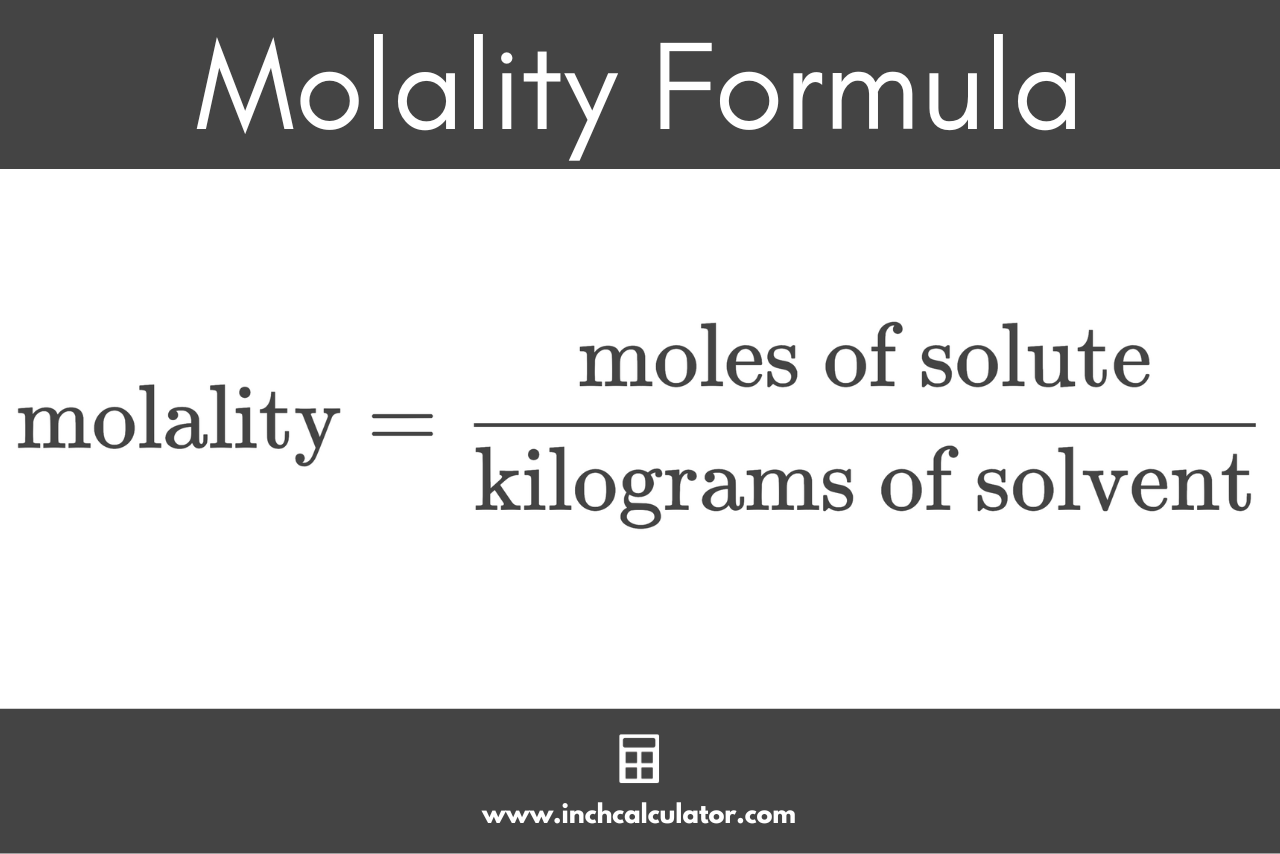# Molality Calculator

Calculate the molality of a solution by entering the amount of solute and the mass of solvent below.

## Molality:

mol/kg
Learn how we calculated this below

## How to Calculate Molality

Molality represents the concentration of a compound in a solution measured as the amount of the substance in moles divided by the mass of the solute in kilograms. Molality is often expressed in moles per kilogram, or mol/kg, and is denoted using the letter m.

First used in 1923, molality is different from molarity in that molality is the moles of the solute in 1 kg of the solvent, while molarity is the moles of the solute in 1 L of the solvent.

If the solvent is water, molality and molarity will be the same since 1 L of water weighs 1 kg.

In general, both of these terms are used to prepare and label standard liquid solutions that are frequently used in a laboratory. Both molality and molarity describe the strength of a chemical in solution; for example, a 2M solution is twice as concentrated as a 1M solution.

### Molality Formula

Since it’s a ratio, you can calculate molality using the following formula:

molality (m) = moles of solute / kilograms of solvent

Thus, the molality m is equal to the amount of substance of solute in moles divided by the mass of solvent in kilograms.For example, let’s calculate the molality of a solution with 3 moles of solute and 2 kilograms of solvent.

molality (m) = 3 moles solute / 2 kg solvent
molality (m) = 3 / 2 = 1.5 mol/kg

In this example, the molality of the solution is equal to 1.5 mol/kg. Notice that it does not matter what the solute and solvent are; the molality would be the same as long as there are 3 moles of that substance in 2 kg of the solvent.

## References

1. Lewis, G. N., Randall, M., Thermodynamics and the Free Energy of Chemical Substances, McGraw-Hill Book Company, Inc., 1923, New York and London, https://books.google.com/books?id=m81AAAAAIAAJ&ots=K6ziAMlj7Y&lr&pg=PR7